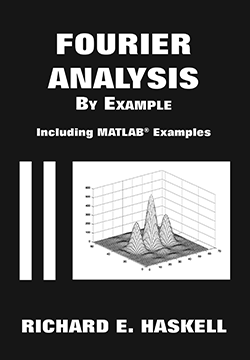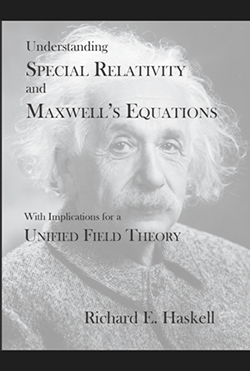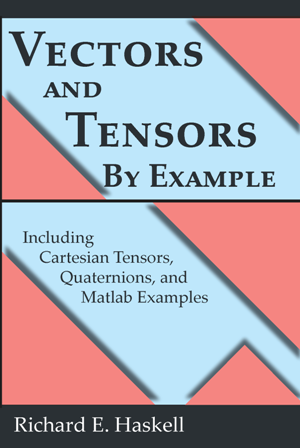## Last Lecture

I retired from Oakland University in 2012, and on April 2, 2012 gave a Last Lecture at Meadow Brook Theatre. You can view this Last Lecture by clicking on the following figure. It lasts about 55 minutes.

Since retiring, I have written the five books shown on this page. Peggy's Discovery is a novel based on many of the ideas I discussed in my Last Lecture. In the novel, Peggy discovers a Theory of Everything. The technical details of everything in the novel are spelled out in the book, Understanding Special Relativity and Maxwell's Equations - With Implications for a Unified Field Theory. I think you will find the Preface to this book interesting. You can read it here. All of the mathematics that you need to understand this book can be found in the book, Vectors and Tensors By Example - Including Cartesian Tensors, Quaternions, and Matlab Examples. The book, Ideas that made your Smartphone - Why the patent system should be abolished, describes many of the thousands of ideas that went into making your smartphone and makes the case that the patent system should be abolished. Fourier Analysis By Example - Including Matlab Examples is my unique approach to understanding Fourier analysis. Click on any of the book covers to order the book from Amazon.## Fourier Analysis By Example

This book begins with examples of calculating the Fourier Transform of various functions and shows that calculating the Fourier Transform of a periodic function leads to the Fourier Series, calculating the Fourier Transform of a sampled function leads to the Discrete-Time Fourier Transform (DTFT), and calculating the Fourier Transform of a sampled, periodic function leads to the Discrete Fourier Transform (DFT). The book includes a detailed derivation of the Fast Fourier Transform (FFT) algorithm for computing the Discrete Fourier Transform. Numerous MATLAB examples of using the FFT include examples of amplitude and frequency modulation as well as binary and quadrature phase shift keying of digital signals. The book shows how the Discrete Cosine Transform (DCT) can be derived from the FFT, and the example of using the two-dimensional DCT as part of the JPEG image compression algorithm is described. Examples from Fourier optics are also included in the book.

## All MATLAB Examples (zip file)## Peggy's Discovery

A book containing a short, simple equation that explains all of physical reality is ignored when published, disappearing from public view for fifty years, until a high-school senior, with the encouragement of her engineering professor uncle, enters college on a quest to understand the nature of physical reality by challenging conventional wisdom.  In the process, she starts a company that revolutionizes higher education, leading her to uncover the secret to a theory of everything.

Richard E. Haskell grew up in New Hampshire and is Emeritus Professor of Engineering at Oakland University in Rochester, Michigan, where he taught electrical and computer engineering for 46 years.  He is the author of over thirty books ranging from plasma dynamics to digital design.  He currently lives in New Hampshire.## Book Highlights:

• Graphical approach to special relativity
• Quaternion representation of the Lorentz transformation
• Relativistic dynamics
• Derivation of Maxwell’s equations from Coulomb’s law and special relativity
• A different approach to gravitational fields
• Details of a little-known unified field theory
• ## Preface## Vectors and Tensors By Example - Including Cartesian Tensors, Quaternions, and Matlab Examples

If you have been confused by vectors, vector calculus, tensor analysis, or quaternions, this book is for you. Packed with examples, including Matlab examples, this book will show you

• How to use Matlab to calculate dot and cross products, and solve linear equations;
• How to prove any vector identity using Cartesian tensors;
• How to derive the expressions for gradient, divergence, Laplacian, and curl in any curvilinear coordinate system;
• How to understand covariant and contravariant components of a vector;
• The meaning of Christoffel symbols in covariant differentiation;
• How to derive the curvature tensor;
• How quaternions can be used to describe vector rotations in 3-D space.